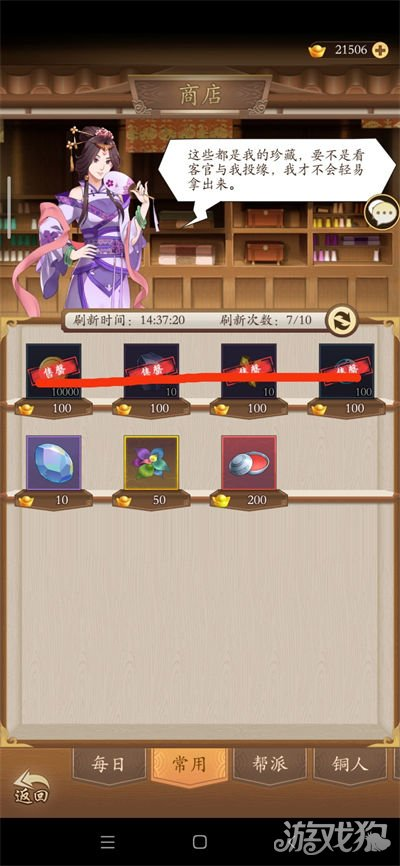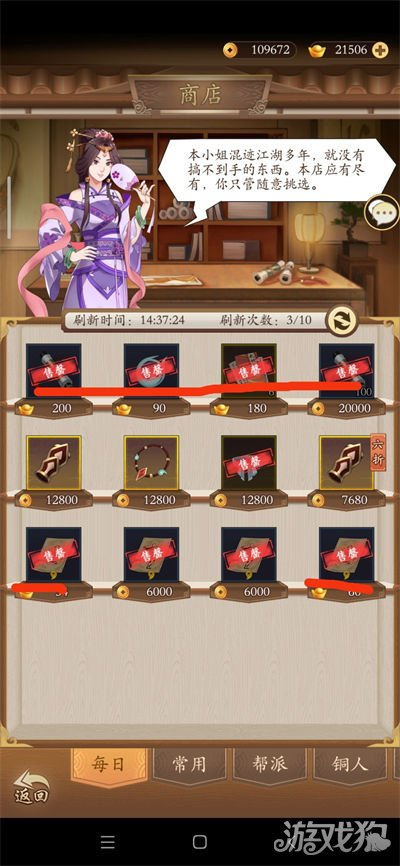# 无名江湖微氪怎么玩 商店购买建议分享

2023-01-03 09:13:00来源：游戏狗整理编辑：卡卡

v3(50+100+100+200+200=650)

v5(再加一次200元宝=850)v3(买77次 77×20/7=220)

v5(买70次 70×20/7=200)

v3(50+300=350)

v5(150+300=450)v5(300+300=600)

(1)商店中每日板块白嫖建议刷新一次，v3刷2次，v5刷3次，一次50元宝，两次50+100=150，三次50+100+150=300，每次刷新必买突破书200+学习点100+武学残卷200+不固定刷新的元宝花费的口诀约100，若刷新出铜币购买的突破书建议买，铜币购买的武学残卷看自己铜币酌情买。总计买买一次约600元宝

v3(150+600×3=1950)

v5(300+600×4=2700)

(2)商店中常用板块刷新次数推荐和上面每日一样。每次刷新必买强化石200+淬英石200+真气值200+铜币100，铜币一次100每次刷新可买5次，铜币缺的话可以多买。总计买一次约700元宝

v3(150+700×3=2250)

v5(300+700×4=3100)

v3(1950+2250=4200)

v5(2700+3100=5800)

(3)帮派商店只买后两个，强化石和淬英石，其余的帮贡全部修炼。铜人商店买自己需要的即可。竞技商店有自己需要的武学就买武学，没有就买普通的券去抽心愿比较靠谱，闭关商店同理。

v3(650+129+220+350+300+4200=5849)

v5(850+129+200+450+600+5800=8029)2.强烈推荐双卡，性价比太高，长期玩的玩 家必备。

3.对于官方来说，多来点福利，多出点元宝获 取活动和玩法### 相关新闻关注游戏狗订阅号